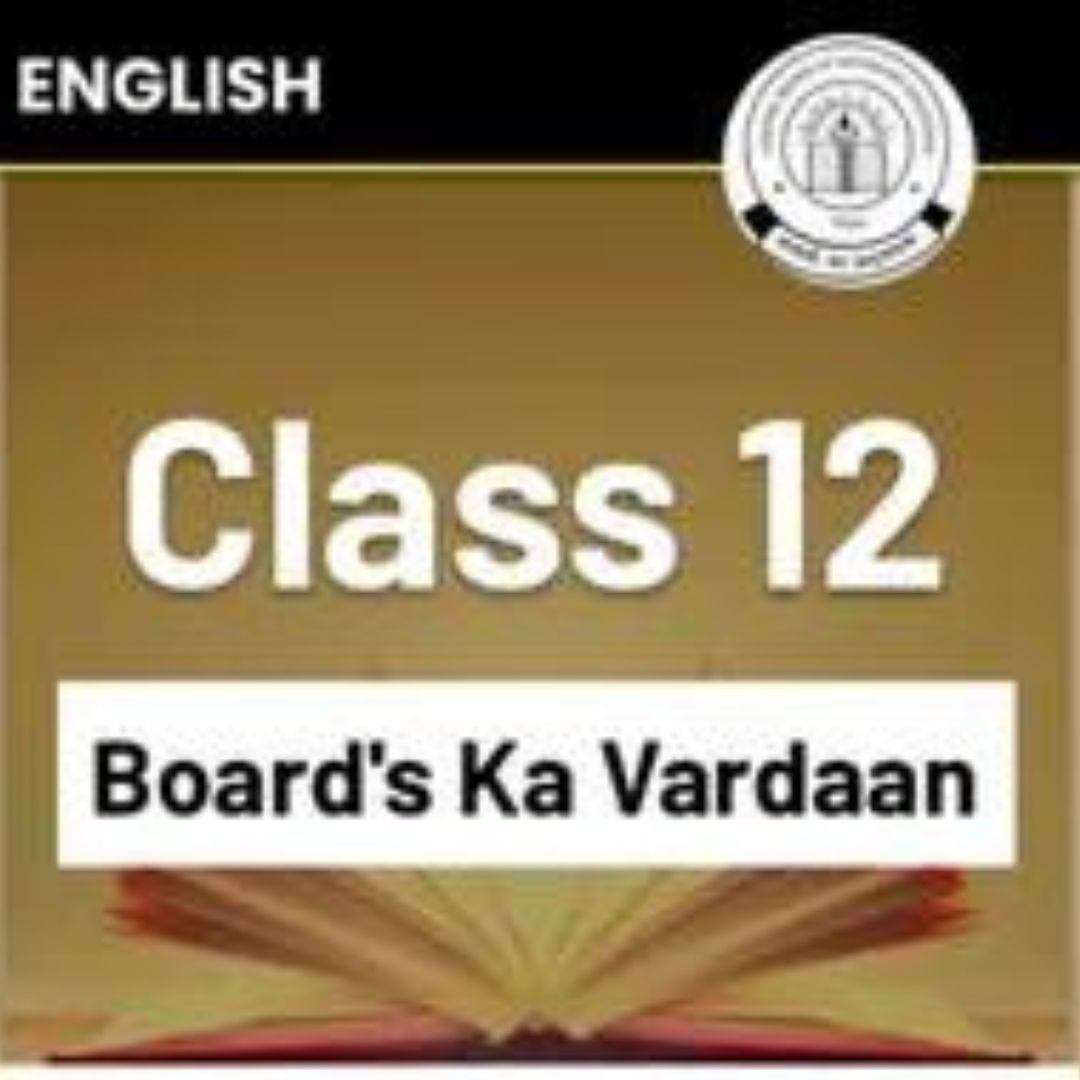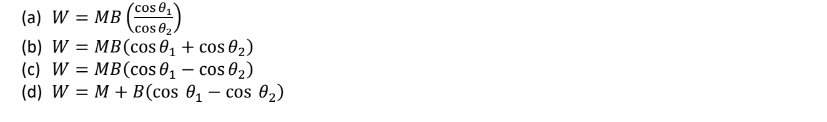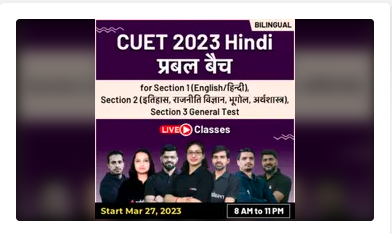Online Tution   »   class 12   »   Class 12 Physics Board Paper 2023

# Class 12 Physics Board Paper 2023 [ Expected ]

## Physics Board Paper 2023

Expected Class 12 Physics Board Paper 2023: The CBSE Class 12 Physics Examination is going to take place on 5th March 2023. So it is a very crucial time to maximize our score in the Class 12 Physics Exam. Students must practice the Model Class 12 Physics Board Paper 2023 for Physics as much as they can, it will beneficiary to face the questions in the Examination hall.

Class 12 Physics Answer Key 2023

Class 12 Physics Sample Paper with Solutions 2023

Class 12 Physics Important Question Chapter wise 2023

Class 12 Physics Previous Year Papers 2023

Class 12 Physics Important MCQs 2023

For the benefit of the class 12 candidates, Our Adda247 Expert faculty prepare the Expected Class 12 Board Physics Exam Paper 2023 which is based on the real Cbse Class 12 Exam pattern. Candidates who are going to take class 12 must take the Class 12 Physics Board Exam Paper 2023## Physics Class 12 Board Expected Question Paper

Adda247’s Class 12 Board Physics Exam Paper 2023, like the official CBSE Class 12 Physics sample paper, comprises five sections with 35 questions for an overall score of 70. Check out the below mark distribution for the Class 12 Board Physics Exam Paper 2023.

• Section A contains 18 MCQ of 1 mark each
• Section B contains seven questions of two marks each,
• Section C contains five questions of three marks each,
• Section D contains three long questions of five marks each
• Section E contains two case study-based questions of 4 marks each.Get Class 12th Boards Ka Vardaan eBook Free Use Code CLASS12

## Class 12 Physics Board Paper 2023-Section A

SECTION A

Q1. When a body is connected to the earth, then electrons from the earth, flow into the body. Then the body is______________.
(a) unchanged
(b) an insulator
(c) positively charged
(d) negatively charged

Q2.A hemisphere is uniformly charged positively. The electric field at a point on a diameter away from the center is directed
(a) perpendicular to the diameter
(b) parallel to the diameter
(c) at an angle tilted towards the diameter
(d) at an angle tilted away from the diameterQ3. Polaroid glasses are used in sunglasses because______________.
(a) They are cheaper
(b) They have a good color
(c) They look fashionable
(d) They reduce the light intensity to half on account of polarization

Q4. Two capacitors of capacitance 6μF and 4μF are put in series across a 120 V battery. What is the potential difference across the 4μF capacitor?
(a) 72 V
(b) 60 V
(c) 48 V

(d) zero
Q5. What is the formula to find the work done in rotating the dipole in a uniform magnetic field from θ1 to θ2 ?Q6. A concave mirror of focal length f produces an image n times the size of the object. If the image is real then
the distance of the object is:
(a) (n − 1)f
(b) (n + 1)f
(c) ( n+1/n ) f
(d) ( n−1/n ) f

Q7. The threshold wavelength for a metal having work function W0 is X. what is the threshold wavelength for the metal having work function 2W0.
(a) 4λ
(b) 2λ
(c) 2/λ
(d) λ/4
Q8. quantity which is not conserved in a nuclear reaction is
(a) momentum
(b) charge
(c) mass
(d) none of these
Q9. A silver wire has a resistance of 2.1 Ω at 27.5 °C, and a resistance of 2.7 Ω at 100 °C. What is the temperature coefficient of resistivity of silver?
(a) 0.0059
(b) 0.0039
(c) 0.0129
(d) 0.0159
Q10.According to Faraday’s law of electromagnetic induction
(a) Electric field is produced by time varying magnetic flux
(b)Magnetic field is produced by time varying electric flux
(c) Magnetic field is associated with a moving charge.
(d)None of these
Q11.The resistance of an ammeter of range 1 ampere is 0.9 Ω. To increase its range to 10 amperes, the required shunt is
(a) 0.1 Ω
(b) 0.01 Ω
(c) 0.9 Ω
(d) 1 ΩQ12. What happens to the inductive reactance when the frequency of the AC supply is increased?
(a) Increases
(b) Decreases
(c) Remains the same
(d) Decreases inversely
Q13. Number of electrons in the valence shell of a pure semiconductor is
(a) 1
(b) 2
(c) 3
(d) 4
Q14 If 13.6eV energy is required to ionise the hydrogen atom, then energy required to remove an electron from n = 2 is
(a) 10.2eV
(b) 0eV
(c) 3.4eV
(d) 6.8eV.
Q15 What is the phase difference between electric and magnetic fields in an electromagnetic wave?
(a) 0
(b) π
(c) π/2
(d) π/4
Q16. Directions: These questions consist of two statements, each printed as Assertion and Reason. While answering these questions, you are required to choose any one of the following four responses.

(a) If both Assertion and Reason are correct and the Reason is a correct explanation of the Assertion.
(b) If both Assertion and Reason are correct but Reason is not a correct explanation of the Assertion.
(c) If the Assertion is correct but Reason is incorrect.
(d) If both the Assertion and Reason are incorrect.
Assertion- two equivalent surfaces can be orthogonal.
Reason- electric field lines are normal to the equipotential surface.

Q17. Directions: These questions consist of two statements, each printed as Assertion and Reason. While answering these questions, you are required to choose any one of the following four responses.
(a) If both Assertion and Reason are correct and the Reason is a correct explanation of the Assertion.
(b) If both Assertion and Reason are correct but Reason is not a correct explanation of the Assertion.
(c) If the Assertion is correct but Reason is incorrect.
(d) If both the Assertion and Reason are incorrect.
Assertion- the poles of magnet cannot be separated by breaking into two pieces.
Reason- the magnetic moment will be reduced to half when a magnet is broken into two equal pieces.

Q18. Directions: These questions consist of two statements, each printed as Assertion and Reason. While answering these questions, you are required to choose any one of the following four responses.
(a)If both Assertion and Reason are correct and the Reason is a correct explanation of the Assertion.
(b)If both Assertion and Reason are correct but Reason is not a correct explanation of the Assertion.
(c) If the Assertion is correct but Reason is incorrect.
(d)If both the Assertion and Reason are incorrect.

Assertion: The binding energy per nucleon, for nuclei with atomic mass number A > 100, decrease with A.
Reason: The forces are weak for heavier nuclei.

Class 12 Physics Previous Year Question Paper with Solution## Class 12 Physics Board Paper 2023- Section B

SECTION B

Q1. What is the reason behind the electrostatic field lines not form closed loop.
Q2. The core of an electromagnet made of ferromagnetic materials. Why?
Q3. For household electrical wiring, one use Cu wire or Al wires. What consideration are kept in mind?
Q4. A glass lens of refractive index 1.45 disappears when immersed in a liquid. What is the value of refractive index of the liquid?
Q5. What is meant by stopping potential in relation to photoelectric effect.
Q6. The mass of a H- atom is less than the sum of the masses of a proton and electron. Why?
Q7. Consider two different hydrogen atoms. The electron in each atom is in an excited state. Is it possible for the electrons to have different energies but the same orbital angular momentum according to the Bohr model?## Expected Class 12 Physics Board Test Paper 2023 -Section C

SECTION C

Q1. What is coherent source of light? How does the resolving power of a compound microscope change when

a) refractive index of medium the object and objective lens increases
b) wavelength of radiation used is increased?

Q2. Prove that the instantaneous rate of change of the activity of a radioactive substance is in proportional to the square of its half-life.
Q3. Write the expression for the force on a charge moving in a magnetic field. By using this equation define the SI unit of the magnetic field.

Q4. The electrostatic force on a small sphere of charge 0.4μC due to another small sphere of charge −0.8μC in air is 0.2 N.

(a) What is the distance between the two spheres?
(b) What is the force on the second sphere due to the first?

Q5. In a single slit diffraction pattern, how does the angular width of central maximum changes when
(i) Slit width is decreased?
(ii) Distance between the slit and screen is increased?
(iii) Light of smaller visible wavelength is used? Justify your answer in each case.## Physics Class 12 Board Paper 2023-Section D

SECTION D

Q1. State and prove Gauss theorem.
Q2. (a) Distinguish between unpolarized light and linearly polarized light. How does one get linearly polarized light with the help of a polaroid?
(b)Explain why the intensity of light coming out of a polaroid does not change irrespective of the orientation of the pass axis of the polaroid.
Q3. (a) The current in the forward bias is known to be more (~mA) than the current in the reverse bias (~μA). What is the reason, then, to operate the photodiode in reverse bias?
(b) how is a light emitting diode fabricated? briefly state it’s working. Write any two important advantages of LEDs over the conventional incandescent low power lamps.## Class 12 Physics Board Paper 2023-Section E

SECTION E

Wavefront is a locus of points which vibratic in same phase. A ray of light is perpendicular to the wavefront. According to Huygens principle, each point of the wavefront is the source of a secondary disturbance and the wavelets connecting from these points spread out in all directions with the speed of wave. The figure shows a surface XY separating two transparent media, medium-l and medium-2. The lines ab and cd represent wavefronts of a light wave travelling in medium- 1 and incident on XY. The lines ef and gh represent wavefronts
of the light wave in medium −2 after refraction.(i) The initial shape of the wavefront of the beam is
(a) planar
(b) convex
(c) concave
(d) convex near the axis and concave near the periphery
(ii) According to Huygens Principle, the surface of constant phase is______.
(a) called an optical ray
(b) called a wave
(c) called a wavefront
(d) always linear in shape
(iii) As the beam enters the medium, it will
(a) travel as a cylindrical beam
(b) diverge
(c) converge
(d) diverge near tile axis and converge near the periphery.
(iv) Which of the following phenomena support the wave theory of light?
(a) Scattering
(b) Interference
(c) Diffraction
(d) DispersionA charged particle moving in a magnetic field experiences a force that is proportional to the strength of the magnetic field, the component of the velocity that is perpendicular to the magnetic field and the charge of the particle. This force is given by F = q(⃗ v ×B ) where q is the electric charge of the particle, v is the instantaneous velocity of the particle, and B is the magnetic field (in tesla). The direction of force is determined by the rules of cross product of two vectors. Force is perpendicular to both velocity and magnetic field. Its direction is same as
(⃗ v ×B ) if q is positive and opposite of (⃗ v ×B ) if q is negative the force is always perpendicular to both the velocity of the particle and the magnetic field that created it. Because the magnetic force is always perpendicular to the motion, the magnetic field can do no work on an isolated charge. It can only do work indirectly, via the electric field generated by a changing magnetic field.

(i) When a magnetic field is applied on a stationary electron, it
(a) remains stationary
(c) moves in the direction of the magnetic field
(d) moves perpendicular to the direction of the magnetic field.

(ii) A proton is projected with a uniform velocity v along the axis of a current carrying solenoid, then
(a) the proton will be accelerated along the axis
(b) the proton path will be circular about the axis
(c) the proton moves along helical path
(d) the proton will continue to move with velocity v along the axis.

(iii) A charged particle experiences magnetic force in the presence of magnetic field. Which of the following statement is correct?
(a) The particle is stationary and magnetic field is perpendicular.
(b) The particle is moving and magnetic field is perpendicular to the velocity
(c) The particle is stationary and magnetic field is parallel
(d) The particle is moving and magnetic field is parallel to velocity
(iv) A charge q moves with a velocity 2 ms1

(iv) A charge q moves with a velocity 2 ms1 along x-axis in a uniform magnetic field ⃗ B= (iˆ + 2jˆ + 3kˆ )T then charge will experience a force

(a) in z ⋅ y plane
(b) along y-axis
(c) along +z axis
(d) along z axis## Class 12 Physics Board Paper 2023- With Solutions

We have also provided you with detailed solutions for Physics Class 12 Board Question paper 2023. So, after solving the Expected Class 12 Physics board Exam paper 2023, students can analyze their scores.Sharing is caring!

## FAQs

### When will CBSE class 12 Physics Exam takes place?

The CBSE Class 12 Physics Examination is going to take place on 5th March 2023.

### How many Sections are there in the Class 12 Board Physics Exam Paper 2023?

The Class 12 Board Physics Exam Paper 2023 comprises five sections with 35 questions for an overall score of 70.

### What is the totals marks of Class 12 Board Physics Exam Paper 2023?

The total mark of the Class 12 Board Physics Exam Paper 2023 is 70 marks

### TOPICS:

[related_posts_view]